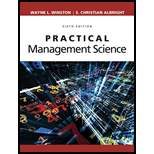# Chemcon has taken over the production of Nasacure from a rival drug company. Chemcon must build a plant to produce Nasacure by the beginning of 2010. Once the plant is built, the plant’s capacity cannot be changed. Each unit sold brings in \$10 in revenue. The fixed cost (in dollars) of producing a plant that can produce x units per year of the drug is 5,000,000 + 10 x . This cost is assumed to be incurred at the end of 2010. In fact, you can assume that all cost and sales cash flows are incurred at the ends of the respective years. If a plant of capacity x is built, the variable cost of producing a unit of Nasacure is 6 − 0.1( x − 1,000,000)/100,000. For example, a plant capacity of 1,100,000 units has a variable cost of \$5.90. Each year a plant operating cost of \$1 per unit of capacity is also incurred. Based on a forecasting sales model from the previous 10 years, Chemcon forecasts that demand in year t , D t , is related to the demand in the previous year, D t −1 , by the equation D t = 67,430 + 0.985 D t −1 + e t where e t is normally distributed with mean 0 and standard deviation 29,320. The demand in 2009 was 1,011,000 units. If demand for a year exceeds production capacity, all demand in excess of plant capacity is lost. If demand is less than capacity, the extra capacity is simply not used. Chemcon wants to determine a capacity level that maximizes expected discounted profits (using a discount rate of 10%) for the time period 2010 through 2019. Use simulation to help it do so.### Practical Management Science

6th Edition
WINSTON + 1 other
Publisher: Cengage,
ISBN: 9781337406659

#### Solutions

Chapter
Section### Practical Management Science

6th Edition
WINSTON + 1 other
Publisher: Cengage,
ISBN: 9781337406659
Chapter 11, Problem 68P
Textbook Problem
5 views

## Chemcon has taken over the production of Nasacure from a rival drug company. Chemcon must build a plant to produce Nasacure by the beginning of 2010. Once the plant is built, the plant’s capacity cannot be changed. Each unit sold brings in \$10 in revenue. The fixed cost (in dollars) of producing a plant that can produce x units per year of the drug is 5,000,000 + 10x. This cost is assumed to be incurred at the end of 2010. In fact, you can assume that all cost and sales cash flows are incurred at the ends of the respective years. If a plant of capacity x is built, the variable cost of producing a unit of Nasacure is 6 − 0.1(x − 1,000,000)/100,000. For example, a plant capacity of 1,100,000 units has a variable cost of \$5.90. Each year a plant operating cost of \$1 per unit of capacity is also incurred. Based on a forecasting sales model from the previous 10 years, Chemcon forecasts that demand in year t, Dt, is related to the demand in the previous year, Dt−1, by the equation Dt = 67,430 + 0.985Dt−1 + et where et is normally distributed with mean 0 and standard deviation 29,320. The demand in 2009 was 1,011,000 units. If demand for a year exceeds production capacity, all demand in excess of plant capacity is lost. If demand is less than capacity, the extra capacity is simply not used. Chemcon wants to determine a capacity level that maximizes expected discounted profits (using a discount rate of 10%) for the time period 2010 through 2019. Use simulation to help it do so.

Summary Introduction

To determine: The capacity-level that maximizes expected discounted profits using @RISK.

Introduction: Simulation model is the digital prototype of the physical model that helps to forecast the performance of the system or model in the real world.

### Explanation of Solution

Formulae to determine the above table:

### Still sussing out bartleby?

Check out a sample textbook solution.

See a sample solution

#### The Solution to Your Study Problems

Bartleby provides explanations to thousands of textbook problems written by our experts, many with advanced degrees!

Get Started

Find more solutions based on key concepts
PAYING OFF CREDIT CARDS Simon recently received a credit card with an 18% nominal interest rate. With the card,...

Fundamentals of Financial Management, Concise Edition (with Thomson ONE - Business School Edition, 1 term (6 months) Printed Access Card) (MindTap Course List)

Why do executives and managers spend more time listening than do workers?

Essentials of Business Communication (MindTap Course List)